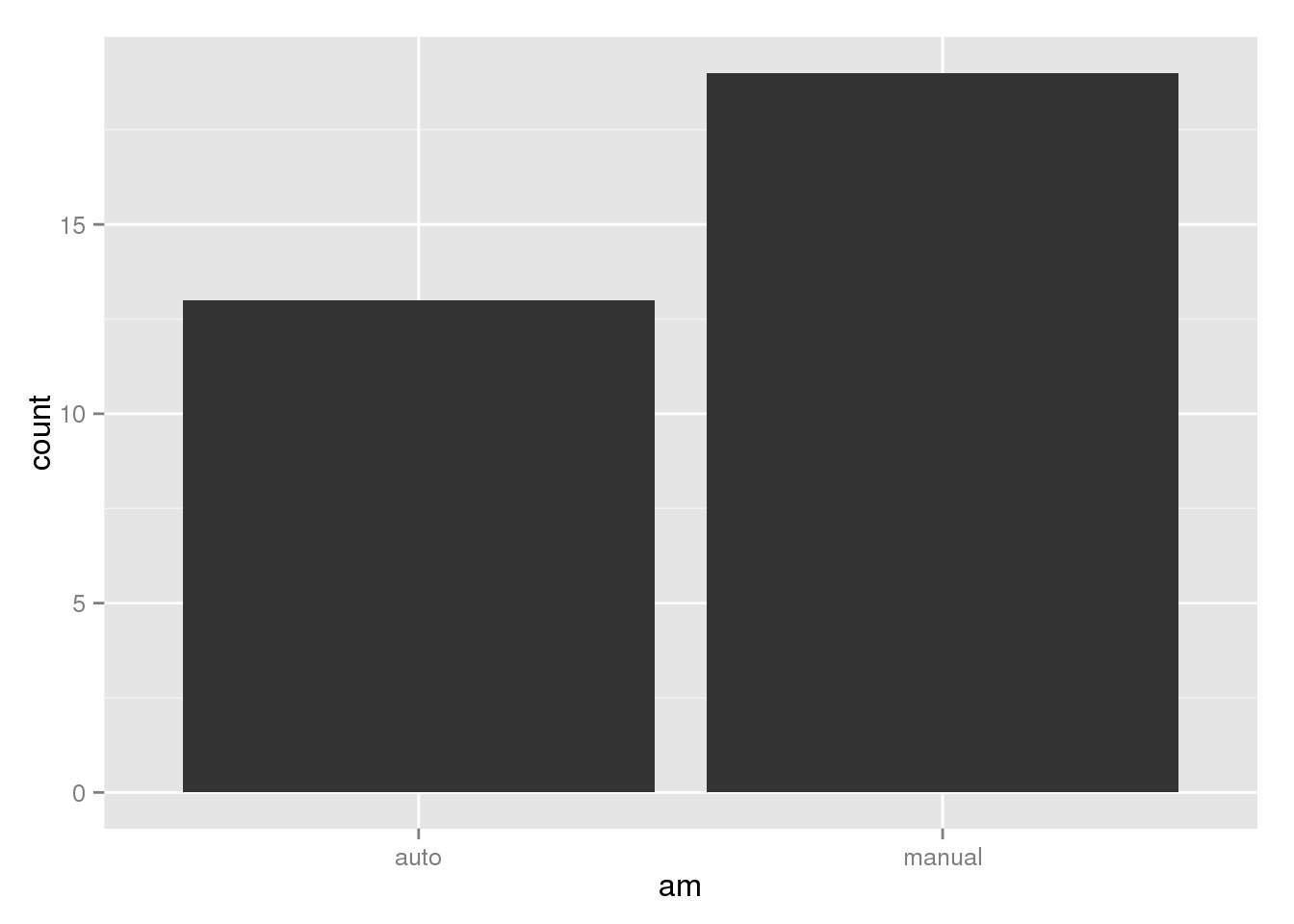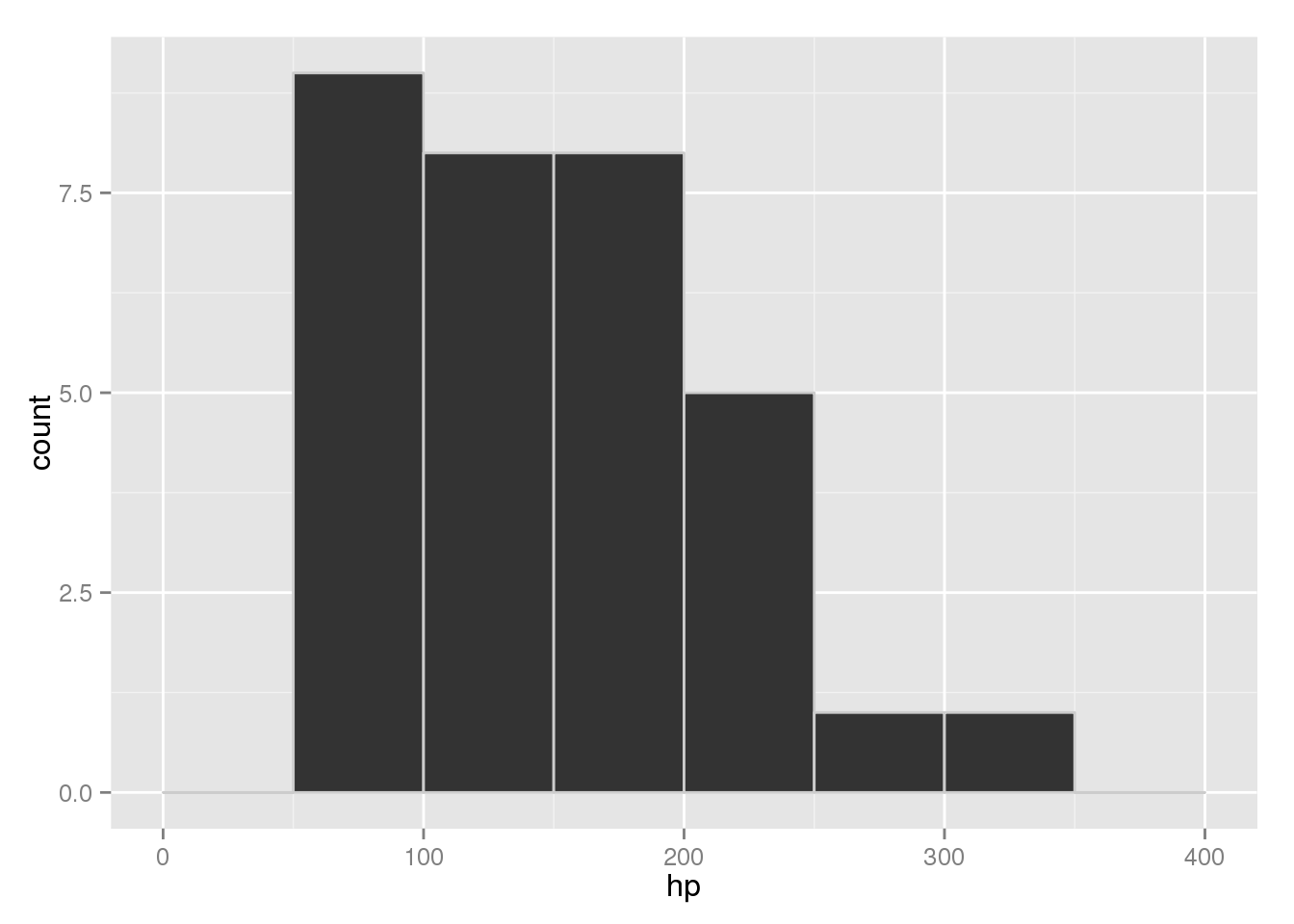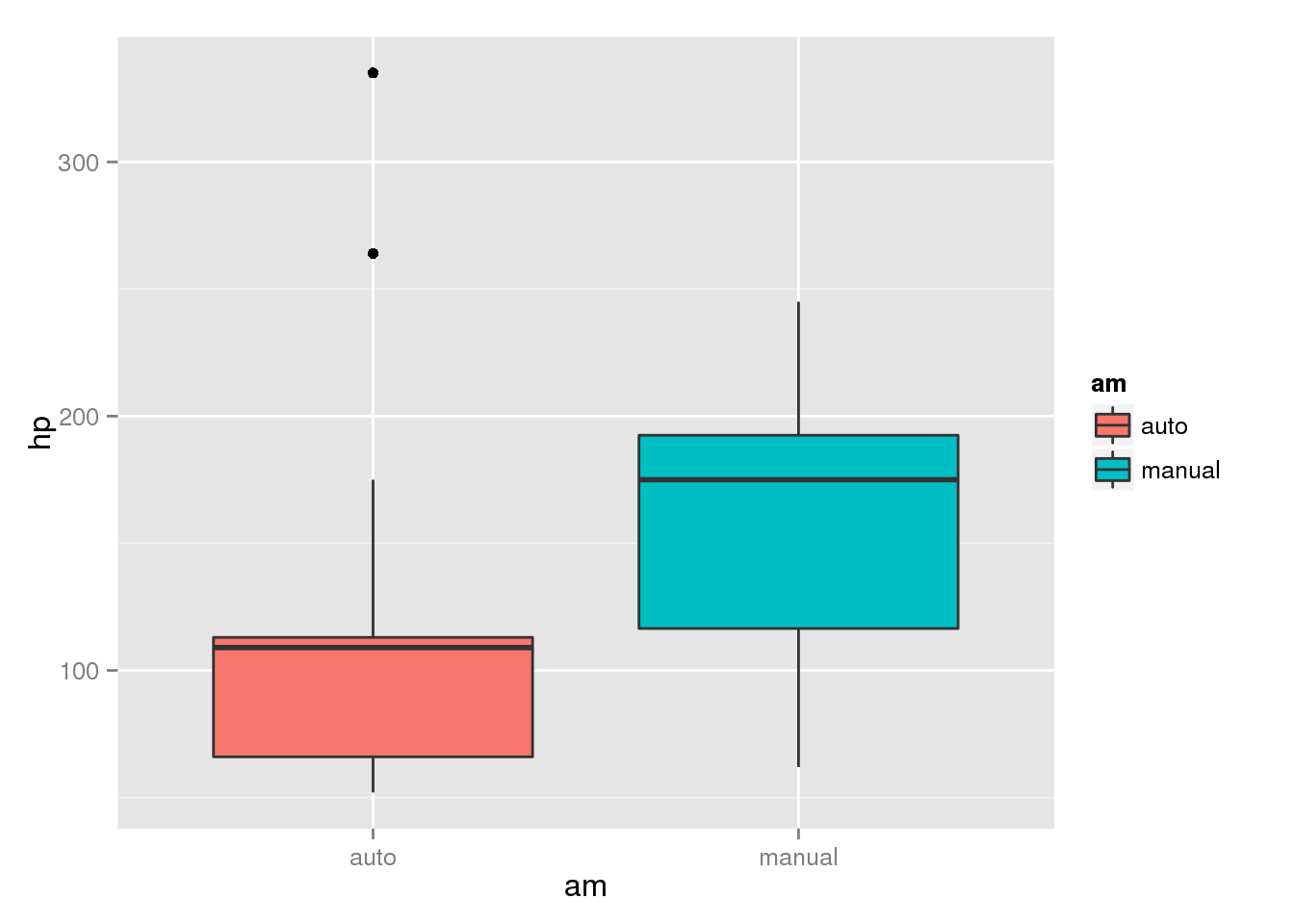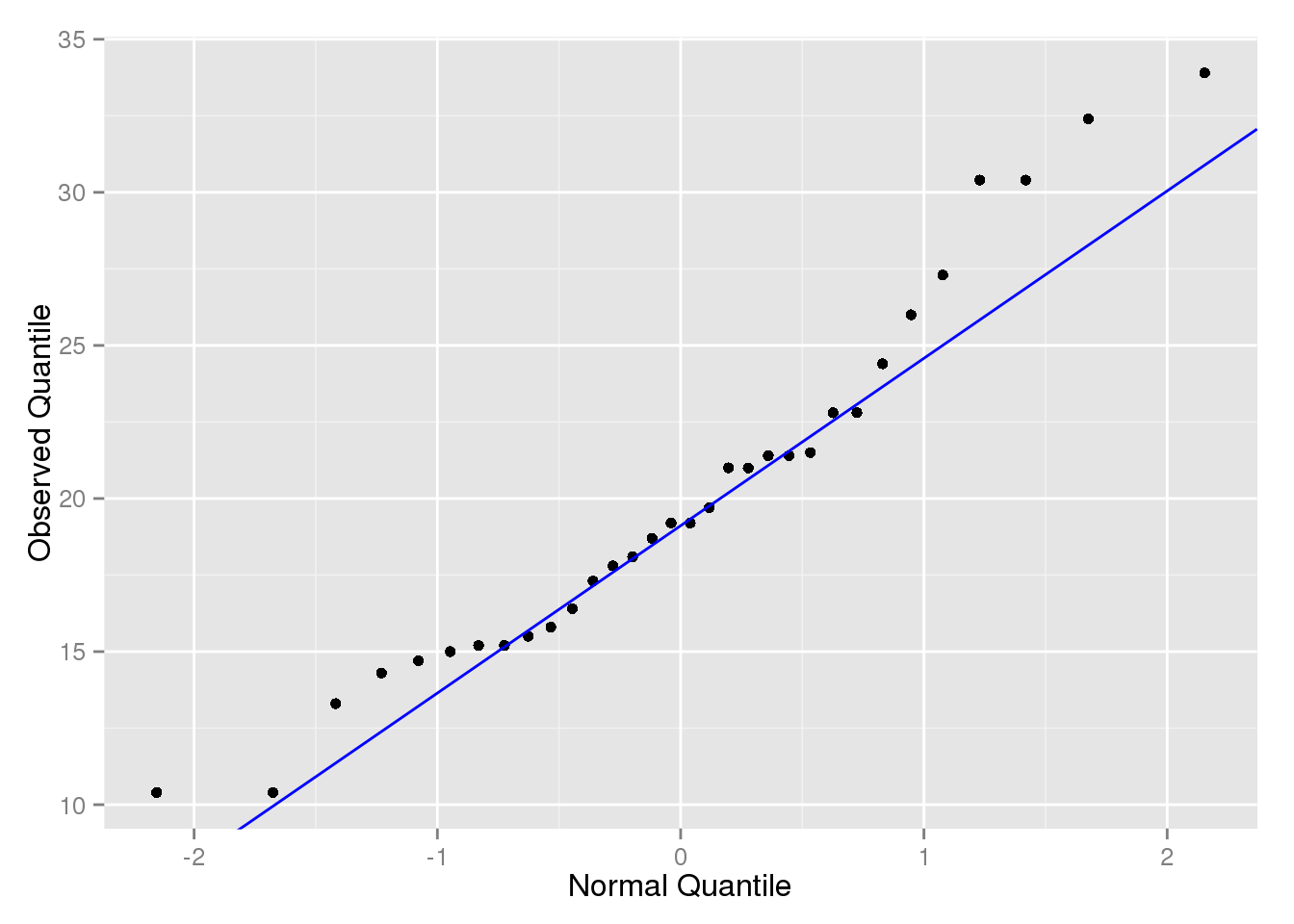## Overview

In this lab, we will examine the Motor Trend Cars data set.

The data was extracted from the 1974 Motor Trend US magazine, and comprises fuel consumption and 10 aspects of automobile design and performance for 32 automobiles (1973–74 models).

Variable Description
mpg Miles/(US) Gallon
cyl Number of Cylinders
disp Displacement (cu.in.)
hp Gross horsepower
wt Weight (lb/1000)
qsec 1/4 mile time
vs V or Straight Configuration Engine
am Transmission Type (auto/manual)

## Load the Data in StatCrunch

A Tab Separated File (.tsv) containing the data can be found here:

1. In StatCrunch, use the menus Data $$\to$$ Load $$\to$$ From File $$\to$$ On the web.
2. Copy and Paste the address given above into WWW Address
3. Click Load File at the bottom of the page.

## Complete the following problems on a separate piece of paper.

Hand in your answers at the end of lab – make sure to include you name and the lab number. Instructions are included for making graphs and finding the necessary statistics for each problem.

Each question will be worth two points, and you can receive partial credit for incorrect answers if your process was correct.

Write your final answer as a sentence and include all steps you used to get there, otherwise you will receive partial credit.

### 1.) List each variable and its type (categorical/quantitative).

library(ggplot2)
data.frame(Variable = colnames(mtcars2)[-1],
Type = c("Numeric", "Categorical", "Numeric", "Numeric", "Numeric",
"Numeric", "Categorical", "Categorical"))
##   Variable        Type
## 1      mpg     Numeric
## 2      cyl Categorical
## 3     disp     Numeric
## 4       hp     Numeric
## 5       wt     Numeric
## 6     qsec     Numeric
## 7       vs Categorical
## 8       am Categorical

counts <- summary(factor(mtcars2$cyl)) n <- nrow(mtcars2) data.frame(Frequency = counts, "Rel. Freq" = counts/n*100) ## Frequency Rel..Freq ## 4 11 34.375 ## 6 7 21.875 ## 8 14 43.750 Most of the cars in the data set have eight cylinders, while six is the least common. ### 3.) Sketch a Bar Plot of am. Which Transmission type is more common? ggplot(mtcars2, aes(x = am)) + geom_bar()Manual cars are more common than automatics in this data set. ### 4.) Make a Histogram of hp (you do not need to sketch it). Describe the shape of the distribution. ggplot(mtcars2, aes(x = hp)) + geom_histogram(binwidth = 50, color = "grey80")The distribution of horsepower is right-skewed and unimodal with no outliers. ### 5.) Which Measure of Center is better for hp? Report it. summary(mtcars2$hp)
##    Min. 1st Qu.  Median    Mean 3rd Qu.    Max.
##    52.0    96.5   123.0   146.7   180.0   335.0

Because the distribution is right-skewed, the median (123 hp) is a more accurate measure of center.

### 6.) Sketch a Side-by-Side Boxplot of hp grouped by am. Describe the differences in the distributions.

ggplot(mtcars2, aes(x = am, y = hp, fill = am)) + geom_boxplot()Manuals have higher horsepower on average and more variation, while there are two automatics with unusually high horsepower.

### 7.) Sketch the QQ Plot of mpg. Is the distribution approximately Normal?

library(car)
gg_qq(mtcars2, "mpg")Because fuel efficiency generally follows the reference line, we can conclude that it is approximately normal.

### 8.) If a certain car had a fuel efficiency of 22 MPG, would it be more rare for an automatic or a manual car? Show your work.

by(mtcars2, mtcars2$am, function(tr) round(data.frame(mean = mean(tr$mpg), sd = sd(tr$mpg)), 2)) ## mtcars2$am: auto
##    mean   sd
## 1 24.39 6.17
## --------------------------------------------------------
## mtcars2\$am: manual
##    mean   sd
## 1 17.15 3.83
(z.auto <- (22 - 24.39)/6.17)
##  -0.3873582
(z.manual <- (22 - 17.15)/3.83)
##  1.266319

22 mpg would be 1.27 standard deviations above the mean for manuals, and 0.39 standard deviations below the mean for automatics. This means that it would be more rare for a manual car to get 22 mpg.

### 9.) Assume that MPG$$\sim N(\mu = 20, \sigma = 6)$$. What is the 90% percentile for the MPG of all cars?

I.e., if $$P(MPG \le x) = 0.9$$, what is $$x$$?

qnorm(.9, mean = 20, sd = 6)
##  27.68931

The 90% of cars have fuel efficiency less than 27.7 mpg.

### 10.) If quarter mile times follow the Normal Model $$N(\mu = 17.85, \sigma = 1.79)$$, how quickly do the middle 75% of cars complete a quarter mile drag race?

I.e., if $$P(l \le QSEC \le u) = 0.75$$, what are $$l$$ and $$u$$?

qnorm(c(.125, .875), mean = 17.85, sd = 1.79)
##  15.79087 19.90913

The middle 75% of cars finish the quarter mile in 15.8-19.9 seconds.